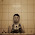### 何謂光圈？#### 再從常用的鏡頭的光圈值來談進光量：

(由左至右，每個光圈值的進光量少一倍)

1， 1.4， 2， 2.8， 4， 5.6， 8， 11， 16， 22， 32

OK，光圈開孔約略成一個圓，而圓面積是這麼算的：半徑^2 × π

#### 接下來要推算出計算下一級光圈值(進光量一半)的公式：

C = R^2 * π，c = r^2 * π

R^2 * π = r^2 * π / 2

R = sqrt(r^2 / 2) = r / sqrt(2) = sqrt(2) * r / 2

D = R * 2 = sqrt(2) * (d/2) / 2 = sqrt(2) * d / 2
D = R * 2 = sqrt(2) * (d/2) / 2 * 2 = sqrt(2) * d / 2

A = f / D = f / ( sqrt(2) * d / 2 ) = f / sqrt(2) / d * 2 = sqrt(2) * (f / d) = sqrt(2) * a

1. 半徑：50 / 2=25

2. 面積：25^2 * π

3. 面積一半：25^2 * π / 2

4. 還原回一半面積的半徑值：sqrt(25^2 * π / 2 / π) = sqrt(25^2 / 2) = sqrt(2) * 25 / 2

5. 再轉成直徑：sqrt(25^2 / 2) * 2 = sqrt(50^2 / 8) * 2 = sqrt(50^2 / 2) = sqrt(2) * 50 / 2

6. 新的光圈值：50 / sqrt(50^2 / 2) = 50 / sqrt(2) / 50 * 2 = sqrt(2) * 50

1 * 根號2 = 1.414 接近 1.4

1.4 * 根號2 = 1.979 接近 2

2 * 根號2 = 2.828 接近 2.8

2.8 * 根號2 = 3.959 接近 4

4 * 根號2 = 5.656 接近 5.6

5.6 * 根號2 = 7.919 接近 8

8 * 根號2 = 11.313 接近 11

11 * 根號2 = 15.556 接近 16

16 * 根號2 = 22.627 接近 22

22 * 根號2 = 31.112 接近 32

《最後再複習一次》

(由左至右，每個光圈值的進光量少一倍)

1， 1.4， 2， 2.8， 4， 5.6， 8， 11， 16， 22， 32

### 留言

1.你算錯囉

R = D / 2，r = r / 2(應該是r = "d" /2，直徑除以二等於半徑)

R = sqrt(r^2 / 2) = r / sqrt(2) = sqrt(2) * r / 2 (上面錯，所以這邊也跟著錯囉)
最後面應該是
R = sqrt(r^2 / 2) = r / sqrt(2) = (d / 2) / sqrt(2)

下面我就自己算了
D=R*2=(d / 2) / sqrt(2) x 2 = d / sqrt(2)
而新的光圈值 A 應該為：(已知：f / d = a)
A = f / D = f / [d / sqrt(2)] = f / d * sqrt(2) = a * sqrt(2)
所以A = a * sqrt(2)

2.謝謝您了，r = d/2 已改正。

不過下面這一行我還沒代入 r = d/2，我是用根式化簡也就是分子分母同乘 sqrt(2) 得到的：
R = sqrt(r^2 / 2) = r / sqrt(2) = sqrt(2) * r / 2

然後在計算 D 的時候，我的 r 是用 d/2 代入的，但是中間少寫了 * 2。
D = R * 2 = sqrt(2) * (d/2) / 2 * 2 = sqrt(2) * d / 2 [其實化簡之後 D 也等於 d/sqrt(2) ]